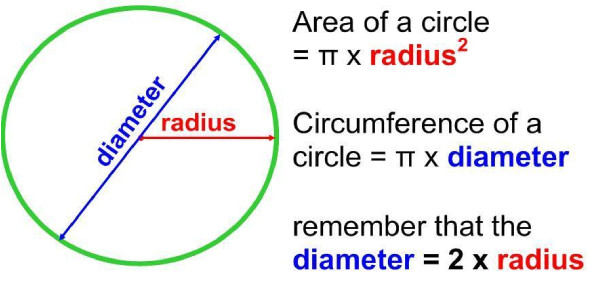# Circumference And Area Of A Circle Quiz

8 Questions | Total Attempts: 341SettingsHow far do you know when it comes to your knowledge in geometry and more specifically to circles. Take this quiz and test yourself in circumference and area related concepts.

Related Topics
• 1.
Round to the nearest tenth. Find the circumference of a circle with a radius of 5.6 meters.
• A.

98.5

• B.

35.2

• C.

36.8

• D.

18.5

• 2.
Round to the nearest tenth. Find the circumference of a circle with a radius of 30.9 centimeters.
• A.

194.1

• B.

2998.1

• C.

97.0

• D.

61.8

• 3.
Round to the nearest tenth. Find the area of a circle with a diameter of 25 yd.
• A.

157.0

• B.

256.3

• C.

1962.5

• D.

503.1

• 4.
Round to the nearest tenth. Find the area of a circle with a diameter of 46.2 cm.
• A.

6702.1

• B.

1587.3

• C.

1675.5

• D.

145.1

• 5.
Round to the nearest tenth. Find the area of a circle with a diameter of 6.8 meters.
• A.

36.3

• B.

36.4

• C.

145.2

• D.

145.1

• 6.
Round to the nearest tenth. A spinkler can spray water 10 feet in all directions. How much area can the sprinkler water?
• A.

62.8 sq.feet

• B.

36.3 sq.feet

• C.

314.0 sq.feet

• D.

98.9 sq.feet

• 7.
Round to the nearest tenth. What is the number of square meters of a half circle whose diameter is 8 m?
• A.

50.2

• B.

45.6

• C.

15.3

• D.

25.1

• 8.
Round to the nearest tenth. Olivia used a stick to draw a circle in the sand. The circle was 4 meters in radius. How many square meters were inside the circle?
• A.

50.2

• B.

25.1

• C.

45.6

• D.

13.6